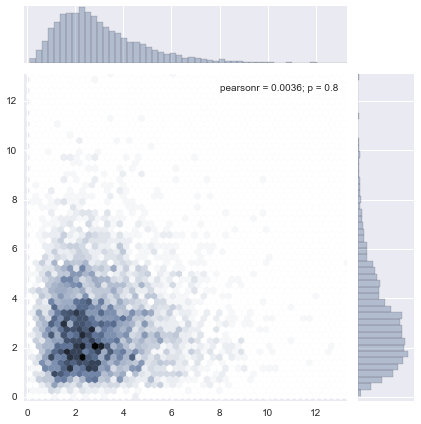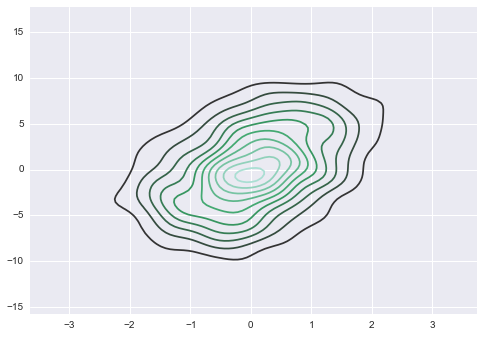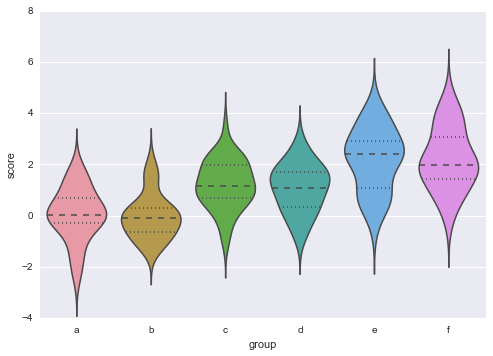# Seaborn¶

• Package by Michael Waskom, a cognitive neuroscience PhD student at Stanford

• Includes themes and hooks for a large class of visualizations

• focuses on visualization for statistical data analysis, including built-in statistical tests

In :
%run talktools
%matplotlib inline

In :
import seaborn as sns
import numpy as np

sns.set_palette("deep", desat=.6)
np.random.seed(9221999)


Now a wide array of beautiful plots can be created with very few lines of code:

In :
from scipy import stats
x, y = stats.gamma(3).rvs((2, 5000))

sns.jointplot(x, y, kind="hex");Seaborn has native support for DataFrame object in the pandas package:

In :
import pandas as pd
data = np.random.multivariate_normal([0, 0], [[1, 2], [2, 20]], size=1000)
data = pd.DataFrame(data, columns=["X", "Y"])

sns.kdeplot(data);Even relatively sophisticated plots can be automatically generated:

In :
y = np.random.randn(200)
g = np.random.choice(list("abcdef"), 200)
for i, l in enumerate("abcdef"):
y[g == l] += i // 2
df = pd.DataFrame(dict(score=y, group=g))

sns.violinplot(df.score, df.group);